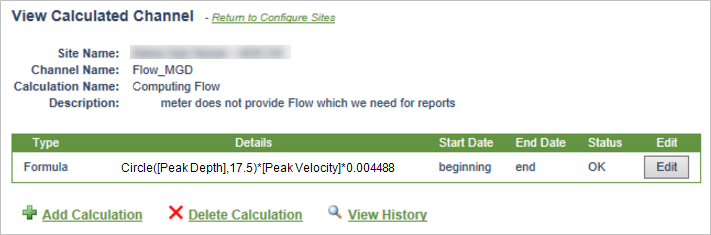# Formula Example: Compute Flow with the Circle Calculation

Some field instruments provide FlowWorks with flow in addition to depth and velocity. However, some instruments are unable to calculate flow on their own. In such a case,  you can use FACE to create a Channel that compiles virtual flow values based on depth and velocity data.

## The process for compiling flow data with the use of Circle is twofold

1. Start by Creating a Calculated Channel. Once complete, you will need to proceed to step 2, which is discussed in this article. The image below shows channel setup for this example. Notice how we set this channel to be expressed in Million Gallons per Day (MGD).1. Configure a FACE formula calculation using Circle, that references both depth and velocity channels, applies the Circle formula, and compiles the resulting virtual flow data in this calculated channel (read below).

This article is a continuation from the article, Create a Calculated Channel. From step one, it is assumed that you are back at the Configure Sites Page, after having completed setup of a new calculated channel.

## 1. Open the calculated channel you just created

1. Use the Choose Site dropdown locate the site that contains the channel with the calculation you wish to use. The page will refresh, revealing channels and calculated channels under this site.
2. Use the Choose Calculated Channel dropdown to select the channel that contains the calculation you wish to configure.
3. Click View Calculated Channel.## 2. Click Create Calculation

This will take you to the page where you will configure the calculation.## 3. Configure the calculated channel

1. Provide a Calculation Name and (optional) Description.
2. Select Formula for Calculation Type.
The bottom of the page will update with drop-down lists to allow you to define the source channel and interval length.
3. Ignore Start Date and End Date.
We want this Channel to calculate Flow for all past, present, and future data.
4. Choose the Source Components.
In this example, we need to select two. For Source Component 1, begin by locating the Site that the depth Channel resides in; then choose the Channel. Next, click  to add a new line for Source Component 2. Locate the Site that the velocity Channel resides in; then choose the Channel.
5. Re-define the Variable Names.
By default, the process has assigned "A" to the depth measurement, and "B" to velocity. You could proceed to use these variable names, however it is good practice to use something that is more likely to make sense later in time. Thus, in this example, we will assign depth with variable "D"; and velocity with variable "V".
6. Leave Choose Lookup Table blank.
7. Define the equation.
We are going to use the Circle function which when given the diameter of a pipe and the depth of the material in that pipe, will return the area cross-section of the pipe occupied by the material. The syntax of this function is: Circle(Depth, Diameter). So if we take this value and multiply it by the velocity, we get flow.
If we go back to just a plot of the Depth and the Velocity from our Site, we see that our measurements for Depth are in inches and the Velocity we are measuring is in feet/second:The conversions needed will be to move the result of the Circle function (which makes no conversions – feed it inches and you get square-inches, feed it feet and you get square-feet) from square-inches to square-feet: 0.006944 square-feet per square-inch.

And to move cubic-feet per second into gallons per day: 0.646316889697 (cubic-feet/per second) * (seconds / day) (million-gallons / cubic-feet).

When we take the result from the Circle function, multiply that by the velocity and then by our two conversion factors, we get: Circle(Depth, Pipe diameter) * Velocity * 0.006944 *0.646316889697 or Circle(Depth, Pipe diameter) * Velocity * 0.004488 = MGD

Thus, in the Define Equation box we will type: Circle(D,17.5)*V*0.004488

The author of this topic is not trained in any way that should be taken as an expert in hydrodynamics. Thus you should use your own constants or prove to yourself that the conversions represented here are valid and more than just looking good.

The final calculation setup looks like this:## 5. Click Process Data Now and WaitFlowWorks creates the new calculated channel immediately. You can use the calculated Flow channel in any of FlowWorks' tools. If we were to graph this new Channel alongside its two source Channels, the resulting graph will look something like this: